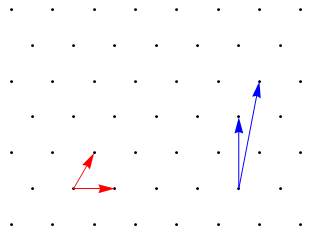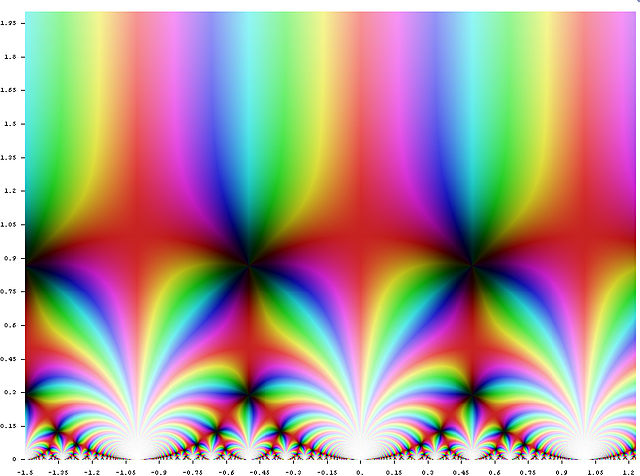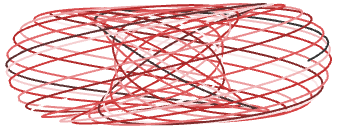# First female Fields medallist

Tomorrow, this year’s Fields medals were awarded*. The four recipients of this prestigious quadrennial accolade have made profound advancements in their respective areas of mathematics, specifically:

• Artur Avila has revolutionised the study of dynamical systems, proving many hitherto open conjectures in the field. This is exciting from both a pure perspective, augmenting our understanding of the ergodic theory of various systems; and from an applied point of view, giving a deeper insight into the behaviour of chaotic systems such as phase transitions.
• Manjul Bhargava‘s research massively generalises Gauss’s composition law, as well as enabling one to enumerate rings of finite rank with a particular discriminant. This has subtle connections to the theory of algebraic curves, including new bounds on the average rank of elliptic curves.
• Martin Hairer made extremely notable discoveries about stochastic partial differential equations, which generalise PDEs by including the effects of random noise. In doing so, he has provided solutions to many new families of SPDEs, several of which have physical interpretations.
• Finally, Maryam Mirzakhani has contributed immensely not only to the geometry of Riemann surfaces and their moduli spaces, but also their dynamics. Incidentally, she is the first female Fields medallist (whilst several great female mathematicians flourished in the twentieth century, including Emmy Noether, Julia Robinson and Ruth Lawrence, the award remained elusive until now), and the remainder of this article is dedicated to her outstandingly brilliant accomplishments.

*International Date Line.Maryam Mirzakhani

Her work is concerned primarily with compact Riemann surfaces of a given genus. From a merely topological perspective, these are multi-holed tori. Riemann surfaces, however, are endowed with a complex structure and therefore a natural metric, which is much deeper and more interesting.

With the exception of the trivial cases of the sphere and torus, these compact Riemann surfaces are quotients of the hyperbolic plane (c.f. Uniformisation Theorem). These quotient spaces are really exciting, and admit an intuitive classification thanks to Thurston’s beautiful theory of orbifolds. Maryam’s early work established a formula for the number of simple closed geodesics on these Riemann surfaces, together with asymptotics for their lengths.

Her next advancements were related to the moduli spaces of Riemann surfaces.

### Moduli spaces

So, what’s a moduli space? It’s probably easiest to explain in the genus-one case, which is simple enough to understand, without being completely trivial. Recall that we can obtain a torus by taking a parallelogram and associating edges. Or equivalently by taking the plane and quotienting by a lattice:In choosing the fundamental parallelogram, we have a huge amount of freedom. Both the red pair and blue pair of vectors above generate the same lattice, and therefore describe geometrically identical tori.

Now, we can consider the four-dimensional parameter space of fundamental parallelograms (i.e. the space of points (x, y, z, w), corresponding to the parallelograms generated by the vectors (x, y) and (z, w)). At the moment this is just plain old$\mathbb{R}^4$, but it is more geometrically meaningful to identify points that describe the same lattice. The resulting quotient space, the moduli space, is a four-dimensional manifold.

Observe that rotating and scaling the two vectors results in geometrically similar lattices (therefore geometrically similar Riemann surfaces). We can consider a further quotient of this four-dimensional space, namely a two-dimensional orbifold. You may recognise this as being the quotient of the upper half-plane by the action of the modular group, or the fundamental region of Klein’s j-function:So, that’s basically what a moduli space is. Maryam discovered a formula for the volume of moduli spaces of a particular genus, resulting in a remarkably elegant new proof of Witten’s conjecture.

### Dynamics

Not merely content with providing a marvellous insightful proof about the geometry of moduli spaces, she decided to investigate their dynamics! Firstly, she demonstrated the ergodicity of Thurston’s ‘earthquake deformation’ of hyperbolic space, which is certainly no mean feat!

Ergodic theory is largely concerned with the topological closure of orbits of points. For example, if we take a geodesic on a torus, it will either form a closed loop (e.g. a torus knot) or an irrational cable:This is a dense subset of the torus, so its topological closure is the torus itself. On more complicated manifolds, such as the moduli spaces considered by Mirzakhani, the topological closures of geodesics can be infinitely intricate fractal structures.

In a similar vein to how holomorphic functions are much more well-behaved than real analytic ones, Mirzakhani settled a long-open conjecture and proved that closures of complex geodesics are always algebraic subvarieties (which are smooth and tame, unlike the pathological fractals that arise in the ergodic theory of real geodesics).

This entry was posted in Uncategorized. Bookmark the permalink.

### 3 Responses to First female Fields medallist

1.Enrique Zeleny says:

Reblogged this on Enrique Zeleny's Blog.

2.Dan Asimov says:

“With the exception of the trivial cases of the sphere and torus, these compact Riemann surfaces are quotients of the hyperbolic plane (c.f. Uniformisation Theorem).”

I don’t think I’d call the case of the torus “trivial”, since these correspond to elliptic curves, which is an extremely deep subject.